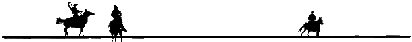Wolverton Mountain PeaceKeepers

August 25-26, 2018

Ariel, WashingtonOregon Old West Shooting Society
 Match Finals with Misses and Procedures
 Tuesday, June 28, 2005 11:44:59 PM 6/25/2005 Page 1 of 2
 Stage 1 Stage 2 Stage 3 Stage 4 Stage 5 Final
 Alias Category Time Time Time Time Time
 Top 5 Overall 26.85 26.2 33.87 33.27 31.55 151.74
 Bart Star Misses: 0 = 0 0 = 0 1 = 5 0 = 0 0 = 0 5
 Penalties: 0 = 0 0 = 0 0 = 0 0 = 0 0 = 0 0
 Bonus: 0 0 0 0 0 0
 26.85 26.2 38.87 33.27 31.55 156.74
 Top 5 Overall 28.57 25.15 32.2 38.46 38.57 162.95
 Sweatin Bullets Misses: 0 = 0 0 = 0 1 = 5 2 = 10 1 = 5 20
 Penalties: 0 = 0 0 = 0 0 = 0 1 = 10 1 = 10 20
 Bonus: 0 0 0 0 0 0
 28.57 25.15 37.2 58.46 53.57 202.95
 Top 5 Overall 35.22 33.89 38.82 40.85 39.28 188.06
 Marshall Bisley Misses: 0 = 0 3 = 15 0 = 0 0 = 0 0 = 0 15
 Penalties: 0 = 0 0 = 0 0 = 0 0 = 0 0 = 0 0
 Bonus: 0 0 0 0 0 0
 35.22 48.89 38.82 40.85 39.28 203.06
 Top 5 Overall 34.01 30.5 31.6 24.87 38.78 159.76
 Buffalo Wings Bryan Misses: 0 = 0 2 = 10 1 = 5 4 = 20 2 = 10 45
 Penalties: 0 = 0 0 = 0 0 = 0 0 = 0 0 = 0 0
 Bonus: 0 0 0 0 0 0
 34.01 40.5 36.6 44.87 48.78 204.76
 Top 5 Overall 48.99 44.16 43.63 45.18 49.35 231.31
 Warmheart Misses: 0 = 0 1 = 5 1 = 5 0 = 0 0 = 0 10
 Penalties: 0 = 0 0 = 0 0 = 0 0 = 0 0 = 0 0
 Bonus: 0 0 0 0 0 0
 48.99 49.16 48.63 45.18 49.35 241.31
 Classic Cowboy 38.96 36.09 41.98 65.99 40.97 223.99
 Redding Renegade Misses: 2 = 10 1 = 5 0 = 0 1 = 5 0 = 0 20
 Penalties: 0 = 0 0 = 0 0 = 0 0 = 0 0 = 0 0
 Bonus: 0 0 0 0 0 0
 48.96 41.09 41.98 70.99 40.97 243.99
 Mens Senior 37.38 36.63 44.03 45.36 42.26 205.66
 Grizzly Wulff Misses: 2 = 10 3 = 15 0 = 0 2 = 10 1 = 5 40
 Penalties: 0 = 0 0 = 0 0 = 0 0 = 0 1 = 10 10
 Bonus: 0 0 0 0 0 0
 47.38 51.63 44.03 55.36 57.26 255.66
 Classic Cowboy 43.84 41.84 48.41 51.05 45.22 230.36
 Buxton Lookout Misses: 1 = 5 1 = 5 3 = 15 2 = 10 1 = 5 40
 Penalties: 0 = 0 0 = 0 0 = 0 0 = 0 0 = 0 0
 Bonus: 0 0 0 0 0 0
 48.84 46.84 63.41 61.05 50.22 270.36
 Mens Senior 48.25 40.45 63.32 49.8 65.35 267.17
 Brazos Bucky Smith Misses: 0 = 0 1 = 5 2 = 10 0 = 0 0 = 0 15
 Penalties: 0 = 0 0 = 0 0 = 0 0 = 0 0 = 0 0
 Bonus: 0 0 0 0 0 0
 48.25 45.45 73.32 49.8 65.35 282.17
 Mens Senior 50.38 47.1 54.85 57.05 57.07 266.45
 Kalapooia Kid Misses: 1 = 5 0 = 0 3 = 15 1 = 5 0 = 0 25
 Penalties: 0 = 0 1 = 10 0 = 0 0 = 0 0 = 0 10
 Bonus: 0 0 0 0 0 0
 55.38 57.1 69.85 62.05 57.07 301.45
 Page 1

Next